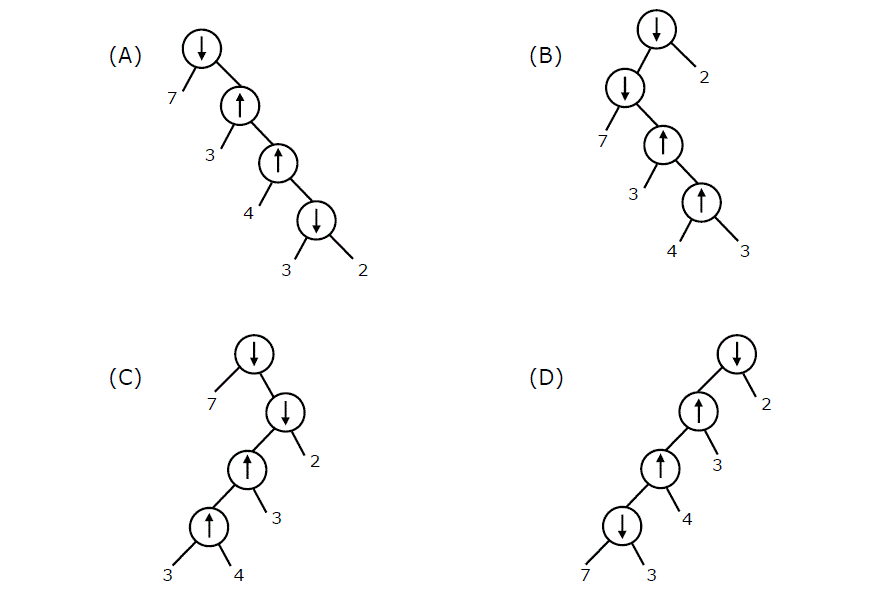Open In App

# Data Structures | Tree Traversals | Question 6

Consider two binary operators ‘‘ and ‘‘ with the precedence of operatorbeing lower than that of theoperator. Operatoris right associative while operatoris left associative. Which one of the following represents the parse tree for expression (73 ­4 ­32)? (GATE CS 2011)(A) A
(B) B
(C) C
(D) D

Explanation: Let us consider the given expression ().
Since the precedence ofis higher, the sub-expression () will be evaluated first. In this sub-expression,would be evaluated first becauseis right to left associative. So the expression is evaluated as. Also, note that among the twooperators, first one is evaluated before the second one because the associativity ofis left to right.